## Using RF Measurement Testbench for IQ-to-RF Converter

Use the RF Measurement Testbench to measure various quantities of an IQ-to-RF converter system. Measurable quantities include cumulative gain, noise figure, and nonlinearity (IP3) values. To open the testbench and measure the quantities, use the RF Budget Analyzer app to create an RF system and then click `Export` > ```Measurement testbench```.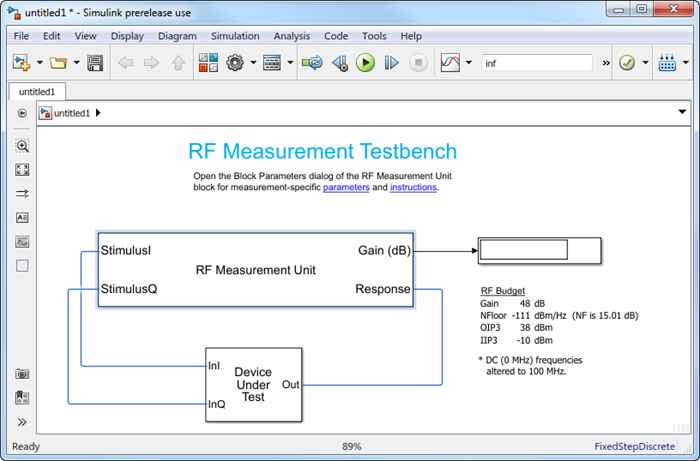The testbench has two subsystems:

• `RF Measurement Unit`

• `Device Under Test`

The testbench display shows the measured output values of the gain, NF (noise figure), IP3 (third-order intercept), and other quantities.

### Device Under Test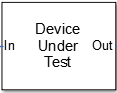The Device Under Test subsystem contains the RF system exported from the app.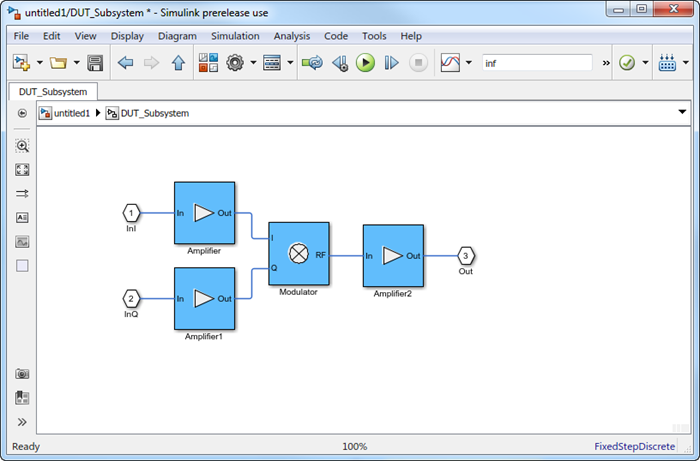### RF Measurement Unit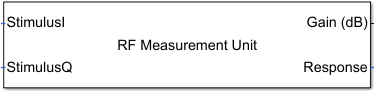The RF Measurement Unit subsystem consists of a Simulink Controller and RF Blockset Circuit Envelope interface. The RF Blockset interface is used as input and output from the DUT.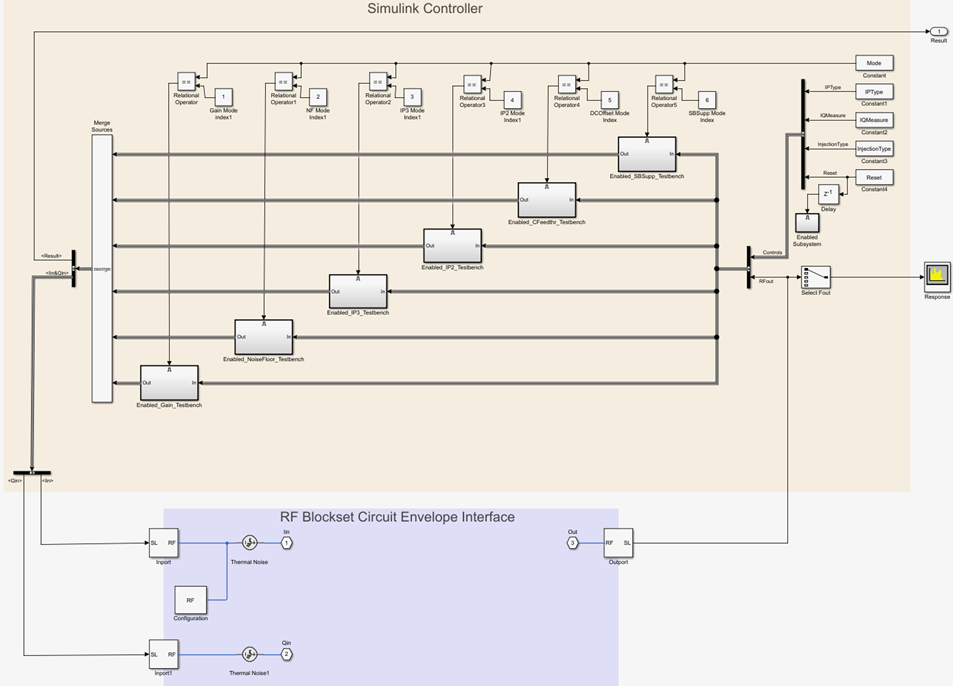### RF Measurement Unit Parameters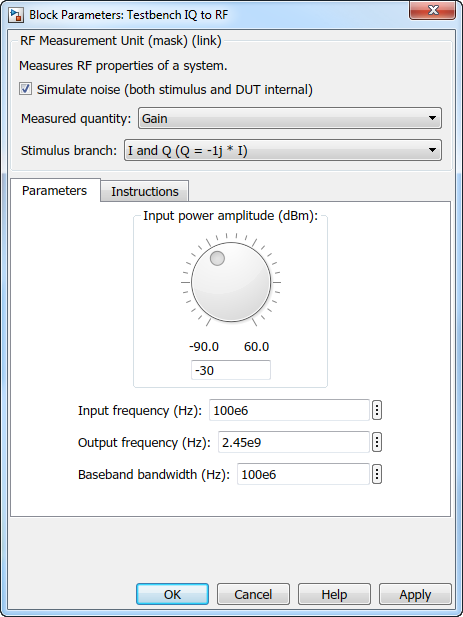• Simulate noise (both stimulus and DUT) — Select this check box to enable noise modeling in the stimulus signal entering the DUT and inside the DUT.

• Measured quantity — Choose the quantity you want to measure:

• `Gain` – Measure the transducer gain of the converter. If you choose only `I` or only `Q` from Stimulus branch, you only see half the value of the measured gain.

• `Noise Floor` – Measure the noise floor value of the converter.

• `IP3` – Measure the output or input third-order intercept (IP3).

• `IP2` – Measure the output or input second-order intercept (IP2).

• `Carrier Feedthrough` – Measure the leakage of carrier tone into the RF spectrum due to imbalances in the in-phase and quadrature phase inputs.

• `Sideband Suppression` – Measure the sideband suppression required for the ideal cancellation of image signals around the RF output signal.

By default, the testbench measures `Gain`. The contents in the Instructions tab changes according to the Measured quantity value.

• IP Type – Choose the type of intercept points (IP) to measure: `Output referred` or ```Input referred```,

By default, the testbench measures `Output referred`. This option is available when you set the Measured quantity to `IP2` or `IP3`.

• Injection Type – Choose the local oscillator (LO) injection for sideband suppression: `Low-side` or `High-side`,

By default, the testbench measures `Low-side`. This option is available when you set the Measured quantity to ```Sideband Suppression```.

• Stimulus branch — Choose the branch you want to use as input stimulus for the measurement:

• `I and Q (Q=-j*I)` — Signal measured is based on a combination of input signals. Quadrature input is same as the in-phase input but is –90 degrees out of phase.

• `I and Q (Q=j*I)` — Signal measured is based on a combination of input signals. Quadrature input is same as the in-phase input but is 90 degrees out of phase.

• `I only(Q=0)` — Signal measured is only the output of the in-phase input signal. Gain measured using this input is only one quarter of the total output signal gain.

• `Q only(I=0)` — Signal verified is only the output of the quadrature input signal. Gain measured using this input is only one quarter of the total output signal gain.

#### Parameters Tab

• Input power amplitude (dBm) — Available input power to the DUT. You can change the input power by manually specifying or by turning the knob. When measuring Carrier Feedthrough, this input field is Input RMS voltage (dBmV), because the feedthrough is measured in voltage units. The specified voltage represents the voltage falling on the input ports of the DUT.

• Input frequency (Hz) — Carrier frequency fed into the I and Q inputs of the DUT. By default, this frequency is by default one bandwidth above DC.

• Output frequency (Hz) — Output frequency to measure the DUT.

• Baseband bandwidth (Hz) — Bandwidth of the input signal.

• Ratio of test tone frequency to baseband bandwidth — Position of the test tones used for IP3 measurements. By default, the value is `1/8`.

This option is only available when you set Measured quantity to `IP2`, `IP3`, and `Carrier Feedthrough`.

#### Instructions Tab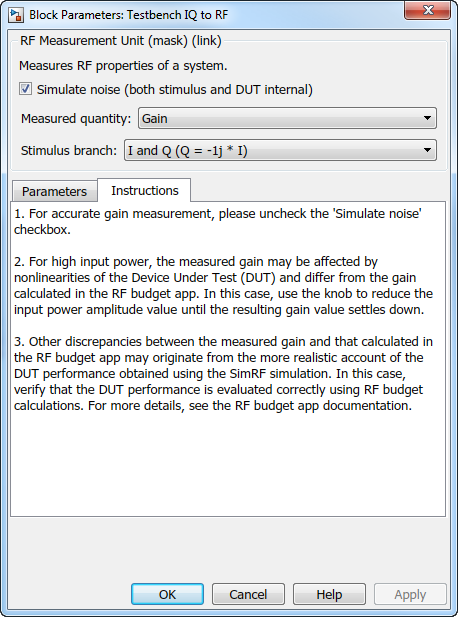Instructions for Gain Measurement

• Clear Simulate noise (both stimulus and DUT) for accurate gain measurement. Select the check box for account for noise.

• Change the Input power amplitude (dBm) or turn the knob to reduce the input power amplitude. For high input power, nonlinearities in the DUT can affect the gain measurements.

Instructions for Noise Floor Measurement

• The testbench measures the spot noise floor calculated. This calculation assumes a frequency-independent system within a given bandwidth. To simulate a frequency-independent system and calculate the correct noise floor value, reduce the baseband bandwidth until this condition is fulfilled. In common RF systems, the bandwidth is reduced below 1 kHz for noise floor testing.

• Change Input power amplitude (dBm) or turn the knob to reduce or increase the input power amplitude. For high input power, nonlinearities in the DUT can affect the noise floor measurements.

Instructions for IP3 and IP2 Measurement

• Clear Simulate noise (both stimulus and DUT) for accurate IP3 and IP2 measurement.

• Change Input power amplitude (dBm) or turn the knob to reduce the input power amplitude. For high input power, higher-order nonlinearities in the DUT can affect the IP3 and IP2 measurements.

Instructions for Carrier Feedthrough Measurement

• Clear Simulate noise (both stimulus and DUT) for accurate IP3 and IP2 measurement.

• Change Input RMS voltage amplitude (dBmV) or turn the knob to reduce the input RMS voltage amplitude. For high input RMS voltage, higher-order nonlinearities in the DUT can affect the carrier feedthrough measurements

• Correct calculation of the carrier feedthrough assumes a frequency-independent system in the frequencies surrounding the test tones. Reduce the frequency separation between the test tones or reduce the baseband bandwidth until this condition is fulfilled. In common RF systems, the bandwidth is reduced below `1 KHz` for carrier feedthrough testing.

Instructions for Sideband Suppression Measurement

• Clear Simulate noise (both stimulus and DUT) for accurate IP3 and IP2 measurement.

• Change Input power amplitude (dBm) or turn the knob to reduce the input power amplitude. For high input power, higher-order nonlinearities in the DUT can affect the sideband suppression measurement.

For all measurements using the testbench, you cannot correct result discrepancies using the RF Budget Analyzer app. The RF Blockset testbench provides true RF circuit simulation that incorporates RF phenomena including saturation and interaction between multiple tones and harmonics in nonlinear devices. These RF phenomena are not incorporated in RF Budget Analyzer, leading to some differences in the values between the testbench and the app.